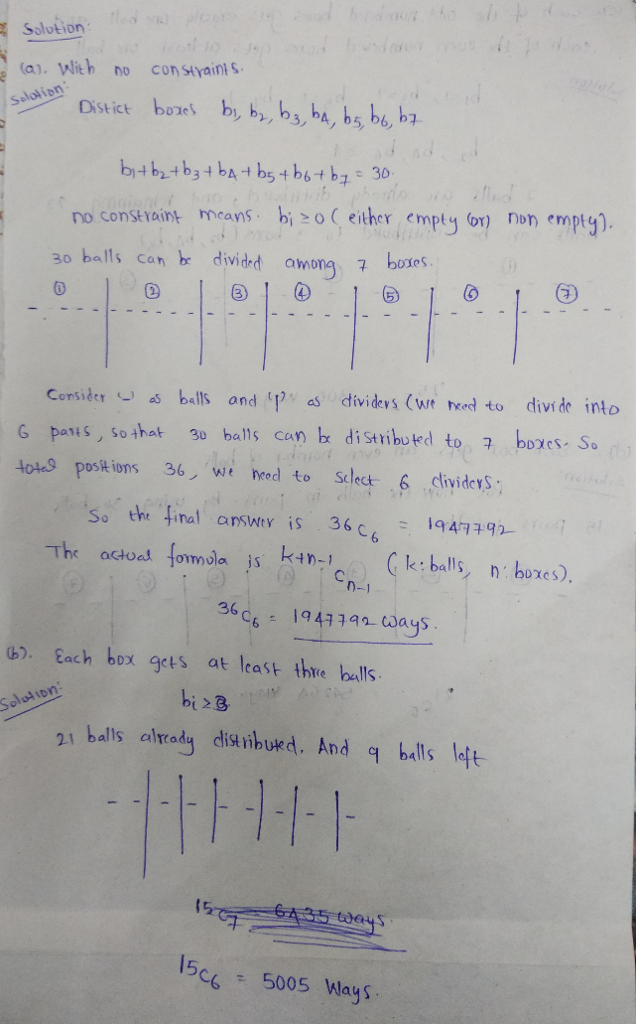# Thread: Discrete Math question

1. ##Discrete Math questionCan someone confirm if im doing this right please?2. ## Re: Discrete Math questionOriginally Posted by inayatCan someone confirm if im doing this right please?
We have one basic rule: Placing $\bf N$ identical objects into $\bf k$ different cells is done in $\bf{\dbinom{N+k-1}{N}}$ ways. So we know the answer for a).

b) If we place three balls into each cell then that means we have nine balls to place into seven cells.

c) place one ball into each box. Now we have twenty three balls left to place into the three even cells: $2,~4,~6$

d) the is done by the tapping method. Tape all balls together two at a time.
Now we have fifteen identical objects to put into seven different cells.
Because adding a series of even numbers is a even number, each of the cells contains an even numbers of balls.